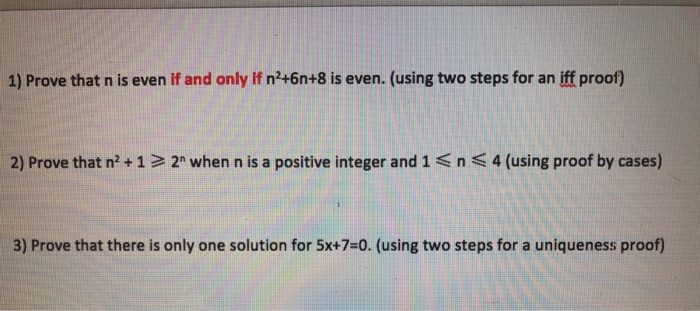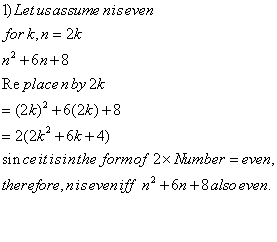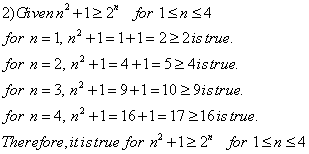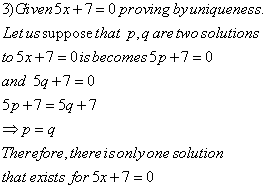# Question & Answer: 1. Prove that n is even if and only if n^2 +6n+8. (Using two steps for an iff proof…..

1. Prove that n is even if and only if n^2 +6n+8. (Using two steps for an iff proof)
2. Prove that n^2+1 >= 2^n when n is positive integer and 1=<n=<4 (using proof cases)
3. Prove that there is only one solution for 5x+7=0. (Using two steps for a uniquness proof)1) Prove that n is even If and only If n’+6n+8 is even. (using two steps for an iff proof) 2) Prove that n2 + 1 > 2n when n is a positive integer and 1 n 4 (using proof by cases) 3) Prove that there is only one solution for 5x+7=0(using two steps for a uniqueness proof)